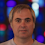## Tuesday, October 04, 2016

### A Second Order Statement true in (R,+) but not (Q,+)

In my last post I asked

Is there a first order statement true in (R,+) but false in (Q,+)

Is there a second order statement true in (R,+) but false in (Q,+)

First order: No.

One reader said it followed from the Lowenheim-Skolem Theorem. I can believe this but don't see how.

One reader said the theory of (Q,+) is complete. True, but would like a reference.

I would prove it by a Duplicator-Spoiler argument.

Second order: Yes

Some readers thought I had < in my language. I do not so I think that examples using < do not work- unless there is someway to express < in my language.

Some readers thought that second order meant you could quantify over functions. My intent was just to be able to quantify over sets.

There exists A,B such that A,B are subgroups with at least two elements that only intersect at 0.

Here is a writeup.

1.The first order equivalence of (Q, +) and (R, +) follows from the Lowenheim-Skolem theorem for the following reason: (Q, +) and (R, +) are both torsion-free divisible abelian groups-- i.e. essentially vector spaces over Q. Thus when you use Lowenheim Skolem to blow (Q, +) up to a model of cardinality |R|, it will be a vector space over Q of the same dimension as (R, +).

2.Clever! I didn't think of using subgroups at all...

3.I think you can define < in second-order (R,+). Sort of. The idea is to consider the second order predicate Pos(X) = X is closed under + and 0 \not\in X and forall x \not= 0, exactly one of x and (-x) are in X. Note here that the maximality condition in the definition of Pos implies that it is closed under multiplication with positive rationals.

Clearly this predicate is true of R^+, but it is also true of other subsets of R too. We can understand their structure. Let B be a basis for R over Q. The elements of B come with "natural" positive or negative assignments, but any predicate X satisfying Pos imposes its own assignment of positive or negative to the basis elements, and moreover, any assignment of positive or negative to the basis elements uniquely determines a set X that satisfies Pos(X).

We can now convert any closed formula \phi in second-order (R,+,<) to an equivalent formula in (R,+) via

forall (X : R -> Prop) (< : R -> R -> Prop), Pos(X) and (x < y iff exists z \in Pos, x+z = y), \phi.

Rod Downey and I had a paper on recursive ordered groups that relied being able to play this sort of game with the order relation, although it wasn't at all framed in this language.

Thoughts?

1.A thought: Isn't it the case that a formula F(x_1,..,x_n) is true in (R,+) iff F(-x_1,..,-x_n) is true in (R,+)? And that holds for first and second order. But then there can be no F(x,y) which is true iff x is less than y (iff -x is greater than -y).

4.Regarding the Löwenheim–Skolem theorem solution: by the LS theorem, there exists a structure (M, +) of cardinality continuum that is elementary equivalent to (Q, +). Note that (M, +) and (R, +) are linear spaces. Since |M| = |R|, (M, +) and (R, +) are isomorphic as linear spaces over Q; thus, (M, +) and (R, +) are isomorphic structures. By transitivity, (Q, +) and (R, +) are elementary equivalent.

5."...Some readers thought that second order meant you could quantify over functions. My intent was just to be able to quantify over sets..." ---> I suspected it, but in this case there is the *widely* used "monadic second order logic" ( only quantification over unary relations :-)

1.... I mean it's more appropriate to use the term "monadic second order" (instead of "second order") in the note to define your problem

6.In the argument using the Lowenheim-Skolem theorem one probably needs more care to argue that the blown up structure (M,+) is indeed elementary equivalent to (R,+). As vector spaces over Q they are not elementary equivalent as (Q,+) is of dimension 1 and (R,+) of infinite dimension.

7.To the last anonymous: the point is that when you blow up (Q, +) to have cardinality |R| it will still be a vector space over Q (because it will still be a torsion-free divisible abelian group) and so it is *isomorphic* to (R, +) and elementary equivalent to (Q, +) because it was chosen as such (using the Lowenheim-Skolem theorem). Of course, as vector spaces Q and R are not isomorphic, but nobody claimed they were.